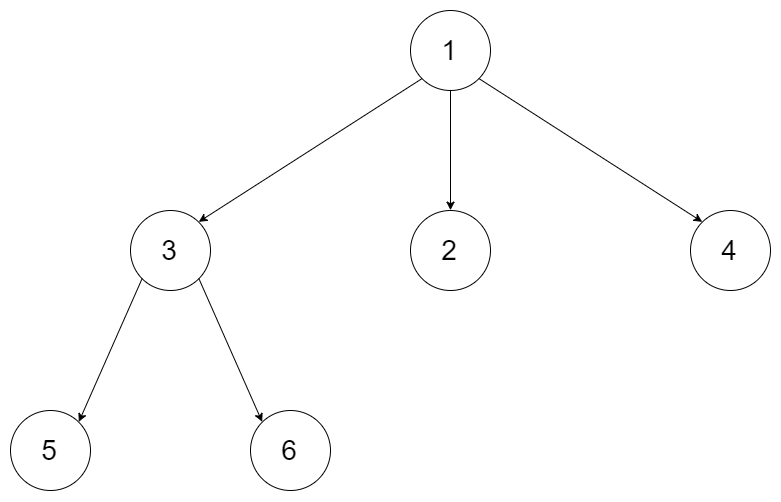Serialize and Deserialize N-ary Tree

Tree, Design

Hard

Serialization is the process of converting a data structure or object into a sequence of bits so that it can be stored in a file or memory buffer, or transmitted across a network connection link to be reconstructed later in the same or another computer environment.

Design an algorithm to serialize and deserialize an N-ary tree. An N-ary tree is a rooted tree in which each node has no more than N children. There is no restriction on how your serialization/deserialization algorithm should work. You just need to ensure that an N-ary tree can be serialized to a string and this string can be deserialized to the original tree structure.

For example, you may serialize the following3-arytreeas[1 [3[5 6] 2 4]]. You do not necessarily need to follow this format, so please be creative and come up with different approaches yourself.

Note:

1. N is in the range of [1, 1000]
2. Do not use class member/global/static variables to store states. Your serialize and deserialize algorithms should be stateless.

Solution

DFS - Recursion (Preferred)

This is a template that can be applied to serialize and deserialize both binary and n-ary trees.

The only difference is that to serialize n-ary tree, we need to append the number of children of a node.

Serialize output with example as input:

1,3,3,2,5,0,6,0,2,0,4,0,

--

/*
// Definition for a Node.
class Node {
public int val;
public List<Node> children;

public Node() {}

public Node(int _val,List<Node> _children) {
val = _val;
children = _children;
}
};
*/
class Codec {

// Encodes a tree to a single string.
public String serialize(Node root) {
StringBuilder sb = new StringBuilder();

serial(sb, root);

// System.out.println(sb.toString());
return sb.toString();
}

private void serial(StringBuilder sb, Node root) {
if (root == null) {
sb.append("#");
sb.append(",");
} else {
sb.append(root.val);
sb.append(",");
if (root.children != null) {
sb.append(root.children.size());
sb.append(",");
for (Node child: root.children) {
serial(sb, child);
}
}
}
}

// Decodes your encoded data to tree.
public Node deserialize(String data) {
Queue<String> queue = new LinkedList<String>(Arrays.asList(data.split(",")));

return buildTree(queue);
}

private Node buildTree(Queue<String> queue) {
String val = queue.poll();
if (val.equals("#")) {
return null;
}

Node root = new Node(Integer.parseInt(val));
int childrenCount = Integer.parseInt(queue.poll());

root.children = new ArrayList<Node>();
for (int i = 0; i <  childrenCount; i++) {
}
return root;
}
}

// Your Codec object will be instantiated and called as such:
// Codec codec = new Codec();
// codec.deserialize(codec.serialize(root));

Pre-order Depth-first search with Stack

example as input

serialize结果

1[3[5[]6[]]2[]4[]]

--

class Codec {

// Encodes a tree to a single string.
public String serialize(Node root) {
if (root == null) {
return "";
}

StringBuilder sb = new StringBuilder();
sb.append(root.val);
sb.append("[");
for (Node child : root.children) {
sb.append(serialize(child));
}
sb.append("]");
return sb.toString();
}

// Decodes your encoded data to tree.
public Node deserialize(String data) {
Node root = null;
Stack<Node> stack = new Stack<>();
int i = 0;

while (i < data.length()) {
int start = i;

// Move pointer forward until we don't find a digit...
while (i < data.length() && Character.isDigit(data.charAt(i))) {
i++;
}

// If we haven't found a digit then we must have found the end of a child list...
if (start == i) {
Node child = stack.pop();
if (stack.isEmpty()) {
root = child;
break;
} else {
// Remove the child from the stack and assign it to the previous node on the stack
}
} else {
Node n = new Node();
n.val = Integer.parseInt(data.substring(start, i));
n.children = new ArrayList<>();
stack.push(n);
}
i++;
}
return root;
}
}

Reference

https://leetcode.com/problems/serialize-and-deserialize-n-ary-tree/discuss/190318/Serialize-and-Deserialize-Binary-and-N-ary-Tree-Summary